# Precalculus : Solve Exponential Decay Problems

## Example Questions

### Example Question #1 : Solve Exponential Decay Problems

The amount of phosphorus present in a sample at a given time is given by the following equation: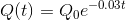Whereis in days,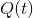is the amount of phosphorus afterdays, and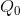is the initial amount of phosphorus at the beginning of the first day. What percent of the initial amount of phosphorus is left afterdays of decay?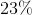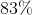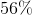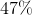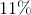Explanation:

The problem asks us for the percent that the amount of phosphorus after t days is of the original amount of phosphorus. If we think of this percentage with respect to the variables present in the equation, we can see that the following fraction expresses the amount of phosphorus after t days as a percentage of the initial amount of phosphorus: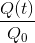So if this is the fraction we want to solve for, we should divide both sides of the equation byto obtain this fraction on the left side of the equation: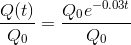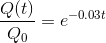We now have the fraction we want to solve for in terms of just one variable,, for which we plug in 25 days to find the percentage of phosphorus left of the initial amount after 25 days: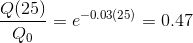So after 25 days of decay, the amount of phosphorus is 47% of the initial amount.

### Example Question #1 : Solve Exponential Decay Problems

The exponential decay of an element is given by the following function: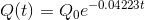Whereis the amount of the element left afterdays, andis the initial amount of the element. If there are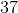kg of the element left afterdays, what was the initial amount of the element?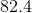kg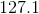kg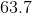kg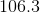kg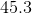kgkg

Explanation:

The problem asks us for the initial amount of the element, so first let's solve our equation for: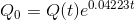The problem tells us that 25 days has passed, which gives us, and it also tells us the amount left after 25 days, which gives us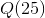. Now that we have our equation for, we can plug in the given values to find the initial amount of the element: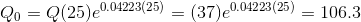kg

### Example Question #1 : Solve Exponential Decay Problems

The exponential decay of an element is given by the function: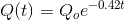In this function,is the amount of the element left afterdays, and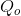is the initial amount of the element. If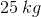of the element is left after seven days, how much of the element was there to begin with, rounded to the nearest kilogram?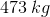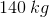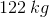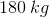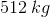Explanation:

To find the initial amount, you must rearrange the equation to solve for:Divide both sides by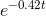: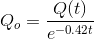Substituting in the values from the problem gives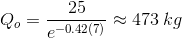### Example Question #2 : Solve Exponential Decay Problems

The exponential decay of an element is given by the function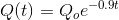In this function,is the amount left afterdays, andis the initial amount of the element. What percent of the element is left after ten days, rounded to the nearest whole percent?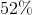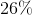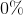Explanation:

To find the final percentage of the element left, we must rearrange the equation to solve for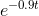: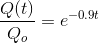Now, using the ten days as, we can solve for the percent of the element left after ten days: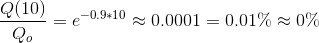### Example Question #1 : Solve Exponential Decay Problems

The exponential decay of an element is given by the function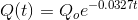whereis the amount of the element afterdays, andis the initial amount of the element. If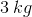of the element are left after four days, how much of the element was there initially, to the nearest tenth of a kilogram?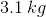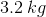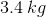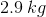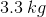Explanation:

To solve for the initial amount, we must use rearrange the equation: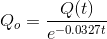We now substitute the values given from the problem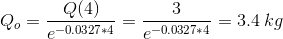### Example Question #3 : Solve Exponential Decay Problems

There were 240mg of caffeine in the discontinued energy drink. The decay rate for caffeine in the human bloodstream is around 0.14. If Jackie drinks this energy drink around 8PM, how much caffeine will still be in her system at midnight?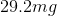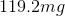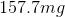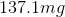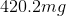Explanation:

Because this is a process taking place in the human body, we should use the exponential decay formula involving e: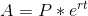where A is the current amount, P is the initial amount, r is the rate of growth/decay, and t is time.

In this case, since the amount of caffeine is decreasing rather than increasing, use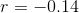. Between 8PM and midnight, 4 hours pass, so use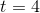. The initial amount of caffeine is given as 240 mg, so use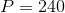.

Now evaluate: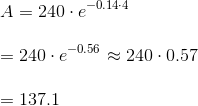### All Precalculus Resources